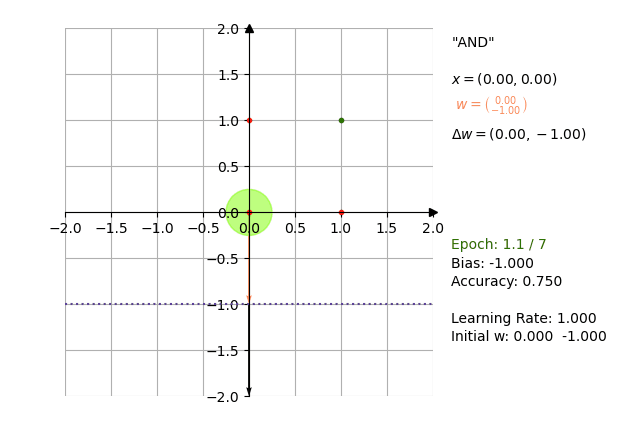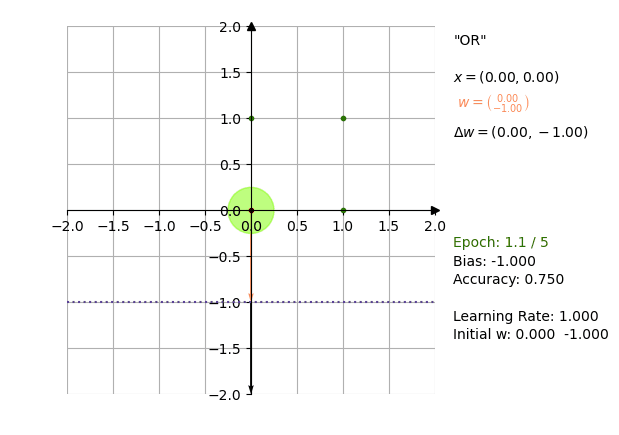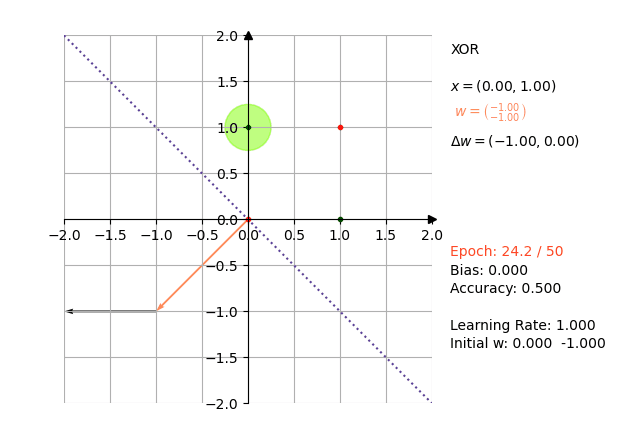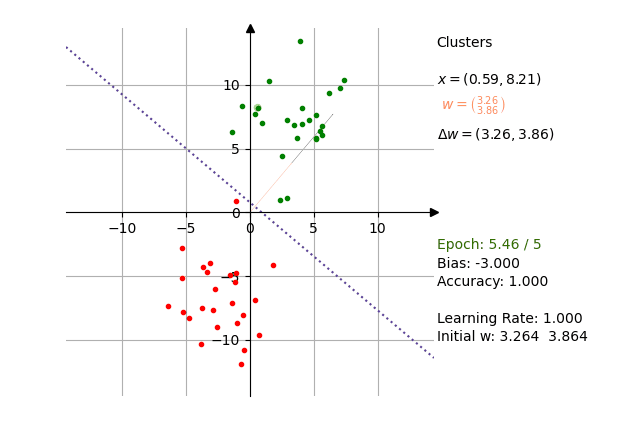# One post tagged with "perceptron"

View All Tags

## Perceptron Function Plotting with Python

I have updated my Perceptron-implementation with a plotting function that allows for visualizing the adjustments of the Perceptron's weight-vector through the epochs.

The source-code can be found at https://github.com/ThorstenSuckow/pylabs.

## Usage​

Create input data and the associated output values. As an example, the following represents the logical AND-function:

import numpy as npfrom Perceptron import Perceptron# inputX = np.array([    [0, 0], [0, 1], [1, 0], [1, 1]])# outputy = np.array([0, 0, 0, 1])

In the next step, the Perceptron is created.

p = Perceptron(50, 0.3)

Once a Perceptron-instance is available, you can pass the input- and output-values to learn():

p.learn(X, y)

and test data with

result = p.test([0, 0])

result holds the computed weight vector if the training data could be separated within the epochs. If that failed, None is returned.

Note: The bias is available with p.bias

A log is available for all steps processed by learn():

for step in p.log:    print(step)

You can pass the log to the PerceptronPlotter which will recreate the computation visually.

## Examples​

### and​

The and-function with a Perceptron.

$A$$B$$A \land B$
111
100
010
000
X = np.array([    [0, 0], [0, 1], [1, 0], [1, 1]])title= "\"AND\""y = np.array([0, 0, 0, 1])p = Perceptron(50)p.learn(X, y)plotter = PerceptronPlotter(p.log, X, y, title)anim = plotter.animate(500)### or​

The or-function with a Perceptron.

$A$$B$$A \lor B$
111
101
011
000
X = np.array([    [0, 0], [0, 1], [1, 0], [1, 1]])title= "\"OR\""y = np.array([0, 1, 1, 1])p = Perceptron(50)p.learn(X, y)plotter = PerceptronPlotter(p.log, X, y, title)anim = plotter.animate(500)### xor​

The xor-function with a Perceptron.

$A$$B$$A \oplus B$
110
101
011
000
X = np.array([    [0, 0], [0, 1], [1, 0], [1, 1]])title= "\"OR\""y = np.array([0, 1, 1, 0])p = Perceptron(50)p.learn(X, y)plotter = PerceptronPlotter(p.log, X, y, title)anim = plotter.animate(500)

With the Perceptron as a linear discriminant function, the algorithm can not properly create a separator for XOR [📖MIN69]. The Plotter shows the Epoch-label marked as red, which tells that the algorithm was not able to find a separator in 50 epochs.### Cluster Example​

The following uses isotropic Gaussian blobs generated by sklearn.datasets.make_blobs. The animate-method is called with an interval of 100 to speed up epoch-runs. The interplay of a larger set of data and the re-adjusting of the separator if accuracy does not reach 1 for a full epoch can be observed nicely.

title = "Clusters"X, y = make_blobs(n_samples=50, n_features=2, centers=2, cluster_std=2.5)p = Perceptron(50)p.learn(X, y)plotter = PerceptronPlotter(p.log, X, y, title)anim = plotter.animate(100)Resources